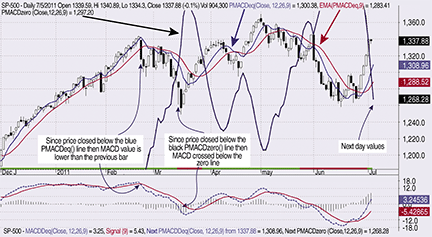INDICATORS

Two Functions That Complement, To Calculate

# Reversing MACD

These two functions complement the MACD and can be used to calculate the price value of a specific MACD level and the price value that will cause the MACD to change direction. Find out how you can apply it to your trading.

Moving average convergence/divergence (MACD) created by Gerald Appel is probably one of the more popular momentum oscillators in use today. It is calculated using two exponential moving averages (EMAs) of different lengths and is the value of the shorter (fast) period MACD less the value of the longer (slow) period EMA. MACD fluctuates above and below the zero value where the moving averages cross.

In Giorgos Siligardos’ article “Reverse Engineering RSI,” he showed that the reverse-engineered relative strength index (RSI) can help determine the following time period’s closing price using the value of the oscillator. And in the article “RSI Bands,” François Bertrand showed overlaying RSI overbought/oversold levels on the price chart.

I will show the calculation of the price value of a specific MACD level and the calculation of the price value that will cause the MACD to change direction. These values in relation to price can then be shown by overlaying them on the price chart. Price charts and code shown here are from the AmiBroker charting package.

Reverse MACD price relationship

```MACDt = MACD(Pt, Xt, Yt)
= EMA (Ptt, Xt) - EMA (Pt, Yt)
```

where:

```Pt is the input series, traditionally a series of closing prices
Xt is the fast period, which usually is 12
Yt is the slow period, which usually is 26

```FIGURE 1: S&P 500 WITH MACD, PMACDEQ(), NINE-PERIOD EMA OF PMACDEQ() AND PMACDZERO() OF THE CLOSING PRICE. Between December 2010 and February 2011, the PMACDeq() line is basically in an uptrend with the closing price oscillating around it. The corresponding MACD is positive, fluctuating up and down almost in a horizontal line, showing that trend may be positive.

…Continued in the January issue of Technical Analysis of Stocks & Commodities